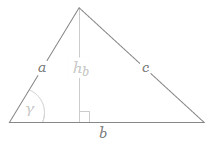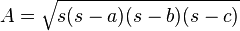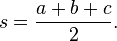## Triangle Area Calculator

Heron's Triangle Area Calculator to find out the Total Triangle Area by using heron's formula.

In geometry, Triangle is a a flat shape with three angles and three straight sides. When three sides of the triangle are all the same length, it is called as equilateral triangle. When two sides of the triangle are the same length, it is called as isosceles. When the triangle whose sides are all of different lengths, it is called as scalene.### Heron's Triangle Area formulawhere s is the semiperimeter of the triangle; that is,### Another Triangle area formula:

S = 1/2 x Base x Height

Thinkcalculator.com provides you helpful and handy calculator resources.